/
/
/
81. An object of mass m and moment of inertia
Not my Question
Flag Content

# Question :   81. An object of mass m and moment of inertia : 2102166

81. An object of mass m and moment of inertia I has rotational kinetic energy KR. Its angular momentum is:

A. 0.5 I/m.

B. (2 IKR)1/2.

C. (2 mKR)1/2.

D. not given above.

82. Two spheres, one with the center core up to r = R/2 hollow and the other solid, have the same mass M and same outer radius R. If they are both rolling at the same linear speed, which one has the greater kinetic energy?

A. The both have the same kinetic energy.

B. The hollow one has the greater kinetic energy.

C. The solid one has the greater kinetic energy.

83. A box slides down a frictionless incline, and a hoop rolls down another incline. Both inclines have the same height, and both the box and the hoop have the same mass. If both objects start from rest, upon reaching the bottom of the incline which one will have the greater kinetic energy and which one will have the greater speed?

A. The box will have both the greater kinetic energy and the greater speed.

B. The hoop will have both the greater kinetic energy and the greater speed.

C. Both will have the same kinetic energy but the hoop will have the greater speed.

D. Both will have the same kinetic energy but the box will have the greater speed.

84. The torque caused by a force F applied at a distance r from the axis of rotation, is given by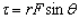, where q is the angle between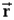and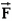. If the magnitudes r and F remain the same, what other angle will produce the same torque as was produced at angle q if q was less than 90??

A.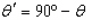B.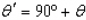C.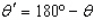D. None of the above.

85. Two equal magnitude forces are in opposite directions and their lines of action are separated by distance d. These two forces are applied to a solid disk, which is mounted on a frictionless axle. If d is half the radius r of the disk, which of the following positions for the forces would give the most torque.

A. One force on a line touching the circumference of the disk, the other on a line halfway to the center.

B. One force on a line at a distance d from the center and the other on a line through the center of the disk.

C. One force on a line at a distance d/2 from the center of the disk, and the other on a line at a distance d/2 on the opposite side of the center of the disk.

D. Since all the above orientations give the same torque, choose this answer.

86. A uniform meter stick balances on a fulcrum placed at the 40 cm mark when a weight W is placed at the 30 cm mark. What is the weight of the meter stick?

A. W

B. 2W

C. W/2

D. 0.4 W

## Solution 5 (1 Ratings )

Solved
Physics 1 Year Ago 238 Views